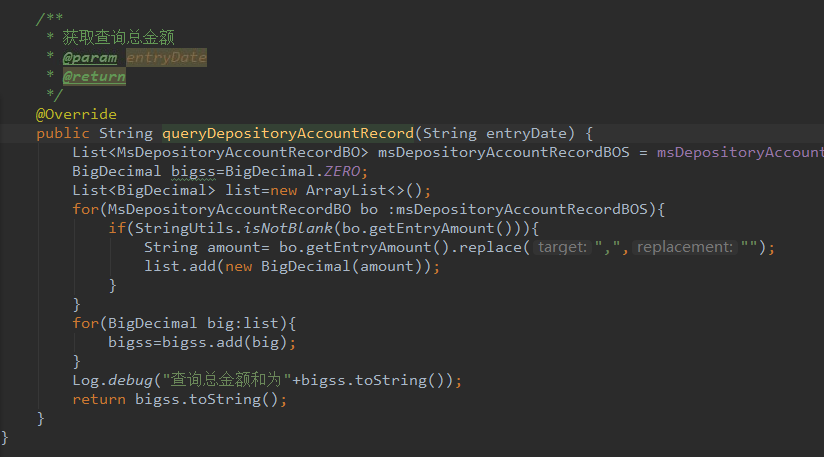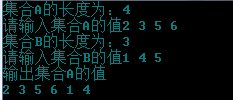• ## 集合相加

千次阅读 2018-08-23 08:40:21
{A} + {B}  HDU - 1412  给你两个集合，要求{A}...=10000),分别表示集合A和集合B的元素个数.后两行分别表示集合A和集合B.每个元素为不超出int范围的整数,每个元素之间有一个空格隔开. Output 针对每组数据输出...
  {A} + {B}
HDU - 1412
给你两个集合，要求{A} + {B}.  注:同一个集合中不会有两个相同的元素.
Input
每组输入数据分为三行,第一行有两个数字n,m(0<n,m<=10000),分别表示集合A和集合B的元素个数.后两行分别表示集合A和集合B.每个元素为不超出int范围的整数,每个元素之间有一个空格隔开.
Output
针对每组数据输出一行数据,表示合并后的集合,要求从小到大输出,每个元素之间有一个空格隔开.
Sample Input
1 2
1
2 3
1 2
1
1 2
Sample Output
1 2 3
1 2
这道题可以用常规方法解决，即把两个集合读入数组中之后再排序，去重，代码如下：
#include <stdio.h>
#include <cstring>
#include <algorithm >

using namespace std;

bool cmp(int x,int y){
return x<y;
}
int main(){
int n,m,l=0,t;
int c;
int d;
while(~scanf("%d %d",&n,&m)){
l=0;
for(int i=0;i<n;i++){
scanf("%d",&c[i]);
}
for(int i=n;i<m+n;i++){
scanf("%d",&c[i]);
}
sort(c,c+m+n,cmp);
for(int i=1;i<n+m;i++){
if(c[i-1]!=c[i])
{
d[l++]=c[i-1];
}
t=l;
d[t]=c[m+n-1];
}
for(int i=0;i<t;i++){
printf("%d ",d[i]);
}
printf("%d\n",d[t]);

}
return 0;
}

不过，我们用集合set来做更加方便，因为set本身就具有排序和去重的特效，只需把两个集合读入set,再输出即可。
代码如下：
#include<stdio.h>
#include<iostream>
#include<set>
#include<vector>
using namespace std;
int main()
{
int n,m,a,b;
set<int> s;
int p;
while(~scanf("%d%d",&n,&m)){
while(n--){
scanf("%d",&a);
s.insert(a);
}
while(m--){
scanf("%d",&b);
s.insert(b);
}
int j=0;
while(!s.empty()){
p[j]=*s.begin();
s.erase(s.begin());
j++;
}
for(int i=0;i<j;i++)
{
if(i!=j-1)
cout<<p[i]<<" ";
else
cout<<p[i];
}
cout<<endl;
}
return 0;
}


展开全文今天因为业务问题需要把两个list加起来，结果相加完后结果为true并不是想要的最终所有值
然后继续尝试重新赋值给一个全新的list，结果还是不行，报类型错误，
错误一
List<Long> list = list1,addAll(list2);
最后经过谷歌得到答案，两个list相加 结果：添加成功就返回true，否则返回 false。
而我们只需要直接拿list1使用就可以了，那就是最终的结果
展开全文java
• //金额List累加注意: BigDecimal里面的格式转换异常 BigDecimal bigss=BigDecimal.ZERO; List&lt;BigDecimal&gt; list=new ArrayList&lt;BigDecimal&gt;(); list.add(new BigDecimal("...

//金额List累加注意: BigDecimal里面的格式转换异常 BigDecimal bigss=BigDecimal.ZERO;  List<BigDecimal> list=new ArrayList<BigDecimal>();  list.add(new BigDecimal("20"));  list.add(new BigDecimal("20"));  list.add(new BigDecimal("20"));  list.add(new BigDecimal("20"));  list.add(new BigDecimal("20"));  for(BigDecimal big:list){      bigss=bigss.add(big);  }  System.out.println("长度是：-----"+list.size());  System.out.println("总金额是：-----"+bigss);展开全文• 主要介绍了java8实现list集合中按照某一个值相加求和,平均值等操作代码，具有很好的参考价值，希望对大家有所帮助。一起跟随小编过来看看吧
• 实现两个集合相加的函数 void MergeList(SqList *l1,SqList l2){ //集合A B 合并 int alength=ListLength(*l1); int blength=ListLength(l2); int num=1,num2=0; for(num;num;num++){ int outnum=GetElem(l2,...
首先顺序表中基本操作
#define MaxSize 100
#define true 1
#define false 0
typedef struct{
int *elem;
int length;
}SqList;
void InitList(SqList *L){
(*L).elem=(int*)malloc(MaxSize*sizeof(int));
(*L).length=0;
}
int ListEmpty(SqList L){
if(L.length==0)
return false;
return true;
}
int GetElem(SqList L,int n){
if(n<1||n>L.length)
return false;
int outnum=L.elem[n-1];
return outnum;
}
int LocateElem(SqList L,int i){
int num=0;
for(num;num<L.length;num++){
if(i==L.elem[num])
return true;
}
return false;
}
void TrverList(SqList L){
int i=0;
for(i;i<L.length;i++)
printf("%d\n",(L.elem[i]));
}
int ListInsert(SqList *L,int i,int e){
if(i>(*L).length+1||i<1)
return false;
int num=(*L).length;
for(num;num>i-1;num--){
(*L).elem[num]=(*L).elem[num-1];
}
(*L).elem[i-1]=e;
(*L).length++;
//return true;
}
int ListLength(SqList L){
return L.length;
}
实现两个集合相加的函数
void MergeList(SqList *l1,SqList l2){
//集合A B 合并
int alength=ListLength(*l1);
int blength=ListLength(l2);
int num=1,num2=0;
for(num;num<=blength;num++){
int outnum=GetElem(l2,num);
if(!LocateElem(*l1,outnum)){
ListInsert(l1,l1->length+1,outnum);
//printf("l.length=%d",l1->length);
}
}
}
主函数
int main()
{
SqList l,n;
SqList *s1=&l;
SqList *s2=&n;
InitList(s1);
InitList(s2);
int i=1,k=1;
int la=0,lb=0;
printf("集合A的长度为：");
scanf("%d",&la);
printf("请输入集合A的值");
for(i;i<=la;i++){
int d=0;
scanf("%d",&d);
ListInsert(s1,i,d);
}
TrverList(*s1);
printf("集合B的长度为：");
scanf("%d",&lb);
printf("请输入集合B的值");
for(k;k<=lb;k++){
int e=0;
scanf("%d",&e);
ListInsert(s2,k,e);
}
TrverList(*s2);
MergeList(s1,*s2);
printf("输出集合A的值\n");
TrverList(*s1);
return 0;
}
测试结果关于GetElem函数：
int GetElem(SqList L,int n，int* e){    if(n<1||n>L.length)        return false;    *e=L.elem[n-1];    return true;
}
这样写的时候输出一直不正确，改了一下方法才能输出。可能是指针问题 翻一下资料在修改
展开全文C语言 数据结构 顺序表
• package first; import java.util.ArrayList; import java.util.List; public class Test { public static List<Double> list = new ArrayList<... public static void main(String[] a...
• 集合处理，属性去重并将数值相加 HashMap<String, SkuDemandItemDTO> map = new HashMap<>(); for (SkuDemandItemDTO item : BomList) {// 遍历原集合 if (map.containsKey(item.getMaterielModel())) ...list java
• 求两个集合的和(并集)、差、交集 set库提供set容器，iterator库提供迭代器，algorithm库提供集合操作(故也可以对其他容器进行集合操作) #include <iostream> #include <set> #include <iterator>...c++
• ## Python 集合与集合运算

万次阅读 多人点赞 2019-01-14 10:32:55
了解 Python 集合: 它们是什么，如何创建它们，何时使用它们，什么是内置函数，以及它们与集合论操作的关系 集合、 列表与元组 列表（list）和元组（tuple）是标准的 Python 数据类型，它们将值存储在一个序列中。...
• 就是一个List集合内有多条数据，如何实现第一条加第二条，第一条加第三条。。。。（然后从第二条开始）第二条加第三条，第二条加第四条。。。。直到结束，将这些加过之后的数据形成一个新的集合 请大神们给点意见，...
• 第一题 ： 统计数组两项相加为给定值的个数 看题很容易 三种解法 第一种用两个for循环暴力破解一样 第二种 一个for循环里通过二分查找-value(两项效果不明显) 第三种 通过排序 在通过排序的信息一遍for循环 下面...算法 二分查找
• 集合: List<User> user = new User(); user .stream().collect(Collectors.summingInt(User::getAge)) 参数类型: summarizingDouble 统计数据(double)状态, 其中包括count min max sum和平均值 ...java
• 给出2个正整数集合A = {pi | 1 &lt;= i &lt;= a}，B = {qj | 1 &lt;= j &lt;= b}和一个正整数s。问题是：使得pi + qj = s的不同的(i, j)对有多少个。  输入  第1行是测试数据的组数n，后面跟着n...
• 给你两个集合，要求{A} + {B}. 注:同一个集合中不会有两个相同的元素. Input 每组输入数据分为三行,第一行有两个数字n,m(0,m),分别表示集合A和集合B的元素个数.后两行分别表示集合A和集合B.每个元素为不超出int范围...
• ## set和集合运算

千次阅读 2019-04-03 14:41:03
set集合 概念：set是可变的、无序的、不重复的元素集合。set的元素及元素里面的元素不能出现不可哈希类型。（即set的元素要求必须可以hash） 常用的不可hash类型有list、set、bytearray set的元素不可索引，...
• =null){ //两个相加链表的当前结点只要有一个不为空就要做加法运算 // int x=(Nowl1!=null)?Nowl1.val:0; //第一个链表的当前节点不为空就取值，否则取0 // int y=(Nowl2!=null)?Nowl2.val:0; // int sum=x+y+carry;...链表
• //最后在把相同部分相加的结果与截取的第三部分相加， //其中会涉及相同部分最大项相加结果大于一的情况，会对此处做处理， //方法为截取的部分最小位直接加一， //加完一后还会涉及最小为是否大于10 public ...java 字符串
• 用C++实现的集合运算（课程设计），实现交，并，补，用类实现，比较简洁。C++
• vue集合添加集合 虚假 (vueuse) Collection of essential Vue Composition API (inspired by react-use) 收集基本的Vue Composition API（受React使用启发） Please [register the Composition API Plugin]...
• ## 两个list相加

千次阅读 2017-04-23 15:31:00
&gt;&gt;&gt; a = ['1','2'] &gt;&gt;&gt; b = ['3','4'] &gt;&gt;&gt; a+b ['1', '2', '3', '4'] &gt;&gt;&gt; a = [1,2] &gt;&...
• ## List遍历相加

千次阅读 2018-06-13 14:03:00
Map<String, Object> map= loanQuotaController.quotaQuery(String.valueOf(id));Long totalQuota=0L;Long quota=0L;if(map.size()!=0){ JSONArray jsonArray = JSONArray.parseArray(map.get("result").toS.....
• ## java集合工具类

千次阅读 2019-05-26 21:47:25
下面小编给大家java的集合工具类：Collections，java提供了一个操作Set、List和Map等集合的工具类：Collections，该工具类里面提供了大量方法对集合元素进行排序、查询和修改等操作，还提供了对集合对象实现同步控制...
• 属性类为int value和String name 在此类下面重写hashCode和equals(Object obj)方法 ...此方法将两个集合为{“value”:“10”,“name“:”ss”}的json格式的返回值中的name相同的value值相加，合并成一个java
• ## List集合累加

千次阅读 2017-09-21 16:11:19
比如说有两个list集合list1和list2，list1有1、2两个元素；list2有3、4两个元素；我们想把这两个集合累加在一起，那么我们可以采用下面的方法，so easy！ package action; import java.util.ArrayList; import java....
• 举个例子： local t1 = {1} local t2 = {2} local t3 = t1 + t2 就会报错： lua: xx.lua:3: attempt to perform arithmetic on a table value (local 't1') 原因： 当Lua试图对两个表进行相加时，先检查两者之一...
• concat() 方法用于连接两个或多个数组。 该方法不会改变现有的数组，而仅仅会返回被连接数组的一个副本。 好了 这样就能返回数据的合并了 88javascript css html5 vue.js
• createorder: function() { var _self = this; var data = []; for (var i = 0; i < _self.msglist.length; i++) { if (_self.msglist[i].medic_sum >... console.log(_self.msglist[i].medic_sum) ......